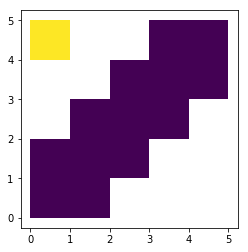In :
import geopandas as gpd

In :
%matplotlib inline

In :
from shapely.geometry import Polygon

polys1 = gpd.GeoSeries([Polygon([(0,0), (2,0), (2,2), (0,2)]),
Polygon([(2,2), (4,2), (4,4), (2,4)])])

polys2 = gpd.GeoSeries([Polygon([(1,1), (3,1), (3,3), (1,3)]),
Polygon([(3,3), (5,3), (5,5), (3,5)]),
Polygon([(0,4), (1,4), (1,5), (0,5)])])

df1 = gpd.GeoDataFrame({'geometry': polys1, 'df1':[1,2]})

df2 = gpd.GeoDataFrame({'geometry': polys2, 'df2':[1,2,3]})

In :
df1

Out:
df1 geometry
0 1 POLYGON ((0 0, 2 0, 2 2, 0 2, 0 0))
1 2 POLYGON ((2 2, 4 2, 4 4, 2 4, 2 2))
In :
df2

Out:
df2 geometry
0 1 POLYGON ((1 1, 3 1, 3 3, 1 3, 1 1))
1 2 POLYGON ((3 3, 5 3, 5 5, 3 5, 3 3))
2 3 POLYGON ((0 4, 1 4, 1 5, 0 5, 0 4))
In :
def explode(gdf):
"""
Will explode the geodataframe's muti-part geometries into single
geometries. Each row containing a multi-part geometry will be split into
multiple rows with single geometries, thereby increasing the vertical size
of the geodataframe. The index of the input geodataframe is no longer
unique and is replaced with a multi-index.

The output geodataframe has an index based on two columns (multi-index)
i.e. 'level_0' (index of input geodataframe) and 'level_1' which is a new
zero-based index for each single part geometry per multi-part geometry

Args:
gdf (gpd.GeoDataFrame) : input geodataframe with multi-geometries

Returns:
gdf (gpd.GeoDataFrame) : exploded geodataframe with each single
geometry as a separate entry in the
geodataframe. The GeoDataFrame has a multi-
index set to columns level_0 and level_1

"""
gs = gdf.explode()
gdf2 = gs.reset_index().rename(columns={0: 'geometry'})
gdf_out = gdf2.merge(gdf.drop('geometry', axis=1), left_on='level_0', right_index=True)
gdf_out = gdf_out.set_index(['level_0', 'level_1']).set_geometry('geometry')
gdf_out.crs = gdf.crs
return gdf_out

In :
df_all = df1.append(df2,ignore_index=True)

In :
df_all

Out:
df1 df2 geometry group
0 1.0 NaN POLYGON ((0 0, 2 0, 2 2, 0 2, 0 0)) 1
1 2.0 NaN POLYGON ((2 2, 4 2, 4 4, 2 4, 2 2)) 1
2 NaN 1.0 POLYGON ((1 1, 3 1, 3 3, 1 3, 1 1)) 1
3 NaN 2.0 POLYGON ((3 3, 5 3, 5 5, 3 5, 3 3)) 1
4 NaN 3.0 POLYGON ((0 4, 1 4, 1 5, 0 5, 0 4)) 1
In [ ]:
df_all["group"] = 1
dissolved = df_all.dissolve(by="group")
gdf_out = explode(dissolved)
gdf_out2 = gdf_out.reset_index()
gdf_out2

In :
gdf_out2

Out:
level_0 level_1 geometry df1 df2
0 1 0 POLYGON ((2 1, 2 0, 0 0, 0 2, 1 2, 1 3, 2 3, 2... 1.0 1.0
1 1 1 POLYGON ((0 4, 0 5, 1 5, 1 4, 0 4)) 1.0 1.0
In :
gdf_out2.plot(column='level_1')

Out:
<matplotlib.axes._subplots.AxesSubplot at 0x7f68952fd8d0>In [ ]: